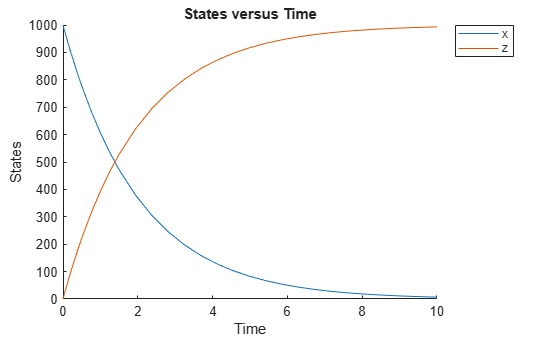# resample

Resample simulation data onto new time vector

## Syntax

``newSimData = resample(simdata)``
``newSimData = resample(simdata,timeVector)``
``newSimData = resample(simdata,timeVector,method)``

## Description

example

````newSimData = resample(simdata)` resamples the simulation data in `simdata` to a common time vector and returns `newSimData`.```

example

````newSimData = resample(simdata,timeVector)` resamples the simulation data to the specified time vector `timeVector`.```

example

````newSimData = resample(simdata,timeVector,method)` resamples the simulation data using the specified interpolation `method`.```

## Examples

collapse all

`sbioloadproject('radiodecay');`

Simulate the model.

```sd = sbiosimulate(m1); sbioplot(sd);```Resample the simulation data between time points from 1 to 5 with the `linear` interpolation method.

```newsd = resample(sd,[1:5],'linear'); sbioplot(newsd);```Change the solver to perform an ensemble run.

```cs = getconfigset(m1); cs.SolverType = 'ssa';```

Perform an ensemble run.

`sdEnsemble = sbioensemblerun(m1,10);`

Resample the ensemble data between time points 1 and 10.

`newsdEnsemble = resample(sdEnsemble,[1:10],'linear');`

Compare the time steps.

`newsdEnsemble(1).Time`
```ans = 10×1 1 2 3 4 5 6 7 8 9 10 ```
`sdEnsemble(1).Time`
```ans = 999×1 0 0.0004 0.0006 0.0047 0.0049 0.0058 0.0105 0.0131 0.0143 0.0144 ⋮ ```

## Input Arguments

collapse all

Simulation data, specified as a `SimData` object or array of `SimData` objects. If you specify an array of `SimData` objects with different time courses, the function selects the time vector from a `SimData` object with the earliest stop time as the common time vector.

Time vector, specified as a numeric vector. The default value `[]` means that the function selects the time vector from `simdata` with the earliest stop time as the common time vector.

If `timeVector` includes time points outside the time interval of the `SimData` objects in `simdata`, `resample` performs extrapolation if the underlying interpolation method supports it. Otherwise, the function returns `NaNs` for those time points. See the help for the MATLAB function corresponding to the interpolation method in use for information on how the function performs the extrapolation.

Interpolation method for resampling, specified as a character vector or string. The valid choices follow.

• `'interp1q'` — Use the `interp1q` function.

• To use the `interp1` function, specify one of the following methods:

• `'nearest'`

• `'linear'`

• `'spline'`

• `'pchip'`

• `'cubic'`

• `'v5cubic'` (same as `'cubic'`)

• `'zoh'` — Specify the zero-order hold.

Note

The `'cubic'` method changed in R2020b to perform cubic convolution. In previous releases, `'cubic'` was the same as `'pchip'`. For details, see `interp1`.

## Output Arguments

collapse all

Resampled simulation data, returned as a `SimData` object or array of `SimData` objects.

## Version History

Introduced in R2007b